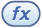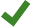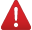# Calculate values using data in table cells

You can create formula or function cells that automatically perform calculations using the data in any cells you select. For example, you can compare values in two cells, calculate the sum or product of cells, and so on. The result of a formula or function appears in the cell where you entered it.

You can also use any of the predefined mathematical functions included with Numbers to create formulas. There are more than 250 functions for applications including statistics, finance, and engineering. Detailed information about each of these functions appears in Function Browser, which appears when you indicate you’re adding a function to a cell (by typing an equal sign in the cell).

## View instant calculations for a range of cells

• Select the column or row (or select the range of cells) whose information you want to view.

Quick calculations for the cells—for example, the sum, average, minimum, maximum, and count—appear at the bottom of the window.

## Quickly sum, average, or count a range of cells

You can quickly find the sum, average, minimum, maximum, count, or product of a range of cells.

1. Do one of the following:

• Select the range of cells you want to include in your function. Numbers chooses a result cell based on your selection.

• Select a cell to display the result of the function. You can’t insert a function in a header cell.

2. Click Functionin the toolbar, then choose a function.

3. If you want to change the range of cells, double-click the result cell. The cells used in the function are highlighted, and the formula editor appears. Do any of the following:

• Resize the selection of cells: Drag the colored dots.

• Move the selection: Drag up or down to change which rows are used, or drag right or left to change which columns are used. Moving the selection doesn’t change the number of cells selected.

4. Clickto save your changes.

If you click, you exit the current cell without saving your changes.

## Insert a function in a cell

You can use any of the 250 predefined mathematical functions included with Numbers in your spreadsheets. The Function Browser includes examples showing how the functions work to help you choose one that suits your needs.

1. Click the cell where you want the result of the function to appear, then type the equal sign (=).

You can also click Functionin the toolbar, then click Create Formula.

The formula editor opens, and the Function Browser appears on the right side of the window. Drag the left side of the formula editor to move it.

2. Type a function name (or terms associated with the function, such as “duration”) in the search field at the top of the Function Browser, or browse the available functions, then double-click the name of the function you want.

The function appears in the formula editor with all of the required and optional arguments for the function.

When you edit a cell that contains a formula, the Smart Cell View at the bottom of the window shows you the formula result, cell reference values, errors, and warnings.

3. Select an argument in the function, then enter a value, or select cells to include in the calculation by doing one of the following:

• Select a cell: Click the cell.

• Select a range of cells across multiple rows and columns: Drag across the range of cells you want to include.

• Add the values of a single column or row: Click the bar at the top of the column or the left of the row—or select all of the cells in the column or row.

4. Press Return or clickin the formula editor when you’re done.

If you click, you exit the current cell without saving your changes.

If there’s an error in your formula,appears in the result cell. Click it to see the error message.

## Edit a function

You can edit an existing function so that it refers to different cells.

1. Double-click the result cell with the formula you want to edit.

The formula editor opens, displaying the functions. Drag the left side of the formula editor to move it.

2. Do any of the following:

• Change the cell references: Select the existing cell addresses you want to change, then select the new cells.

• Remove cell references: Place the insertion point within the argument area for the function, select the unwanted cell references, then press Delete.

• Add more cell references: Place the insertion point within the argument area for the function, then select the new cells you want to add.

3. Press Return or clickin the formula editor when you’re done.

If you click, you exit the current cell without saving your changes.

## Add, subtract, multiply, or divide values

You can create simple or complex arithmetic formulas to perform calculations on the values in your tables.

1. Click the cell where you want the result to appear, then type the equal sign (=).

The formula editor opens. Drag the left side of the formula editor to move it.

2. Select a cell to use as the first argument in your formula, or type a value (for example, a number such as 0 or 5.20).

3. Type an arithmetic operator (for example, +, -, *, or /), then select a cell to use as the next argument in your formula, or type a value.

By default, Numbers inserts a + between cell references.

4. Continue adding operators and arguments until your formula is complete, then press Return or clickin the formula editor when you’re done.

If you click, you exit the current cell without saving your changes.

## Compare values

You can use comparison operators to check whether the values in two cells are equal, or if one value is greater or less than the other. To do this, you must set up a statement within a cell—for example A1 > A2, meaning the value in cell A1 is greater than the value in cell A2. The result of the comparison operator is expressed as “true” or “false.”

1. Click the cell where you want the comparison result to appear, then type the equal sign (=).

The formula editor opens. Drag the left side of the formula editor to move it.

2. Select a cell whose value you want to compare, or type a value to compare.

3. Type a comparison operator (>, >=, =, <>, <, or <=), then select a cell whose value you want to compare, or type a static value to compare.

4. Press Return or clickin the formula editor when you’re done.

If you click, you exit the current cell without saving your changes.

## Preserve row or column addresses in formulas

You can set row and column references in a formula to be absolute so that you can use the same formula elsewhere in your spreadsheet without changing the cell references.

If you don’t preserve the row or column references, then when you move the formula (by cutting and pasting, or by adding new rows and columns), the references are adjusted relative to the formula’s new location.

1. Double-click the result cell with the formula you want to edit.

The formula editor opens, displaying the functions. Drag the left side of the formula editor to move it.

2. Click the triangle on the token representing the cell range you want to preserve.

3. Select Preserve Row or Preserve Column for the beginning or ending addresses of the selected range.

4. Press Return or clickin the formula editor when you’re done.

If you click, you exit the current cell without saving your changes.

## Use MathType to add mathematical expressions and equations

If you have MathType 6.7d or later installed, you can use it to include mathematical expressions and equations in your spreadsheet.

1. Click where you want the equation to appear.

2. Choose Insert > MathType Equation (from the Insert menu at the top of your computer screen).

3. Type or paste your equation in the field provided.

See MathType Help for instructions on using the MathType tools.

4. To save your equation, choose File > Close and Return to Numbers (from the MathType File menu at the top of your computer screen), then click Yes in the window that appears.

The equation appears as an image at the insertion point in your spreadsheet. To edit the equation, double-click it to open MathType again.

You can change the appearance of the equation just as you can other images in your spreadsheet.